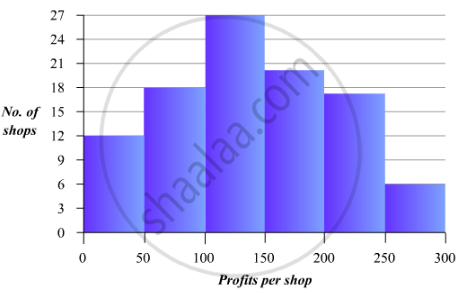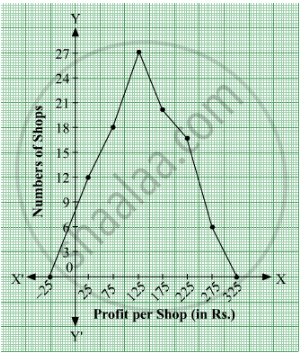# The Monthly Profits (In Rs.) of 100 Shops Are Distributed as Follows:Draw a Histogram for the Data and Show the Frequency Polygon for It. - Mathematics

The monthly profits (in Rs.) of 100 shops are distributed as follows:

 Profits per shop: 0-50 50-100 100-50 150-200 200-250 250-300 No. shops: 12 18 27 20 17 6

Draw a histogram for the data and show the frequency polygon for it.

#### Solution

To represent the given data by a histogram, we first draw horizontal and vertical axes. Let us consider that the horizontal and vertical axes represent the class-intyervals and the frequencies of the class-intervals respectively.

The given data is a continuous grouped frequency distribution with equal class-intervals. Construct rectangles with class-intervals as bases and respective frequencies as heights. The scale for horizontal axis may not be same as the scale for vertical axis. Let us take one vertical division is equal to 3 shops.

The heights of the different rectangles are as following

1. The height of the rectangle corresponding to the class-interval 0-50 is   12/3 = 4 big divisions.

2. The height of the rectangle corresponding to the class-interval 50-100 is 18/3 = 6 big divisions.

3. The height of the rectangle corresponding to the class-interval 100-150 is  27/3 = 9 big divisions.

4. The height of the rectangle corresponding to the class-interval 150-200 is  20/3 = 6.67 big divisions.

5. The height of the rectangle corresponding to the class-interval 200-250 is 17/3 = 5.67 big divisions.

6. The height of the rectangle corresponding to the class-interval 250-300 is  6/3 =2 big divisions.

The histogram of the given data is as follows:For frquency polygon, first we will obtain the class marks as given in the following table.

 Profits per shop Class Marks Number of shops 0-50 25 12 50-100 75 18 100-150 125 27 150-200 175 20 200-250 225 17 250-300 275 6

We plot the points (25, 12), (75, 18), (125, 27), (175, 20), (225, 17) and (275, 6).
Now, we join the plotted points by line segments . The end points (25, 12) and (275, 6) are joined to the mid-points (−25, 0) and (325, 0) respectively of imagined class-intervals to obtain the frequency polygon.

The frequency polygon of the given data is as follows:Concept: Graphical Representation of Data
Is there an error in this question or solution?

#### APPEARS IN

RD Sharma Mathematics for Class 9
Chapter 23 Graphical Representation of Statistical Data
Exercise 23.3 | Q 8 | Page 42

Share